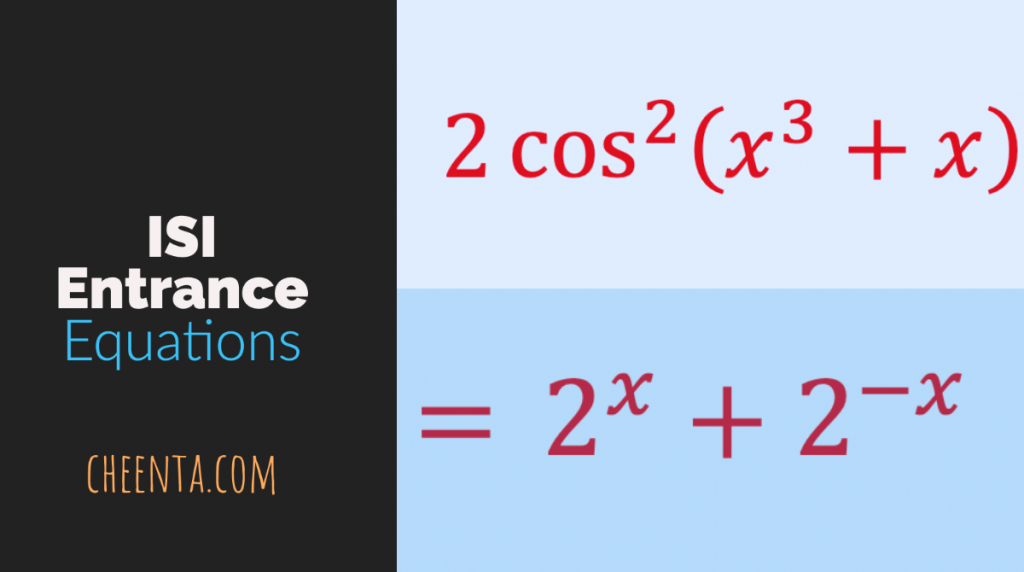How Cheenta works to ensure student success?
Explore the Back-Story

# Bijection Principle Problem | ISI Entrance TOMATO Obj 22Here is a video solution for a Problem based on Bijection Principle. This is an Objective question 22 from TOMATO for ISI Entrance. Watch and Learn!

Here goes the question…

Given that: x+y+z=10, where x, y and z are natural numbers. How many such solutions are possible for this equation?

We will recommend you to try the problem yourself.

Done?

Let’s see the solution in the video below:

## Related Program

Here is a video solution for a Problem based on Bijection Principle. This is an Objective question 22 from TOMATO for ISI Entrance. Watch and Learn!

Here goes the question…

Given that: x+y+z=10, where x, y and z are natural numbers. How many such solutions are possible for this equation?

We will recommend you to try the problem yourself.

Done?

Let’s see the solution in the video below:

## Related Program

This site uses Akismet to reduce spam. Learn how your comment data is processed.

### Knowledge Partner